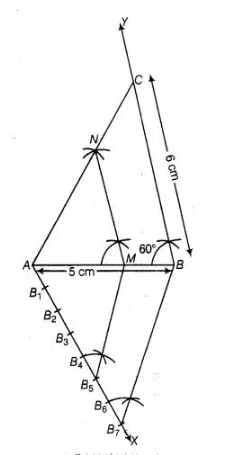# Draw a ΔABC in which AB = 5 cm,

Question:

Draw a $\triangle \mathrm{ABC}$ in which $\mathrm{AB}=5 \mathrm{~cm}, \mathrm{BC}=6 \mathrm{~cm}$ and $\angle \mathrm{ABC}=60^{\circ}$.

Draw a ΔABC in which AB = 5 cm,Construct a triangle similar to ABC with scale factor $\frac{5}{7}$ Justify the construction.

Solution:

Steps of construction

1. Draw a line segment $A B=5 \mathrm{~cm}$.

2. From point $B$, draw $\angle A B Y=60^{\circ}$ on which take $B C=6 \mathrm{~cm}$.

3. Join $A C, \triangle A B C$ is the required triangle.

4. From $A$, draw any ray $A X$ downwards making an acute angle.

5. Mark 7 points $B_{1}, B_{2}, B_{3}, B_{4}, B_{5}, B_{6}$ and $B_{7}$ on $A X$. such that $A B_{1}=B_{1} B_{2}=B_{2} B_{3}$

$=B_{3} B_{4}=B_{4} B_{5}=B_{5} B_{6}=B_{6} B_{7} .$

6. Join $B, B$ and from $B_{5}$ draw $B_{5} M \| B, B$ intersecting $A B$ at $M$.

7. From point $M$ draw $M N \| B C$ intersecting $A C$ at $N$. Then, $\triangle A M N$ is the required triangle whose sides are equal to $\frac{5}{7}$ of the corresponding sides of the $\triangle A B C$.Justifleation

Here, $B_{5} M \| B_{7} B$ (by construction)

$\therefore$ $\frac{A M}{M B}=\frac{5}{2}$

Now, $\frac{A B}{A M}=\frac{A M+M B}{A M}$

$=1+\frac{M B}{A M}=1+\frac{2}{5}=\frac{7}{5}$

Also, $M N \| B C$

$\therefore \quad \Delta A M N \sim \triangle A B C$

Therefore, $\quad \frac{A M}{A B}=\frac{A N}{A C}=\frac{N M}{B C}=\frac{5}{7}$